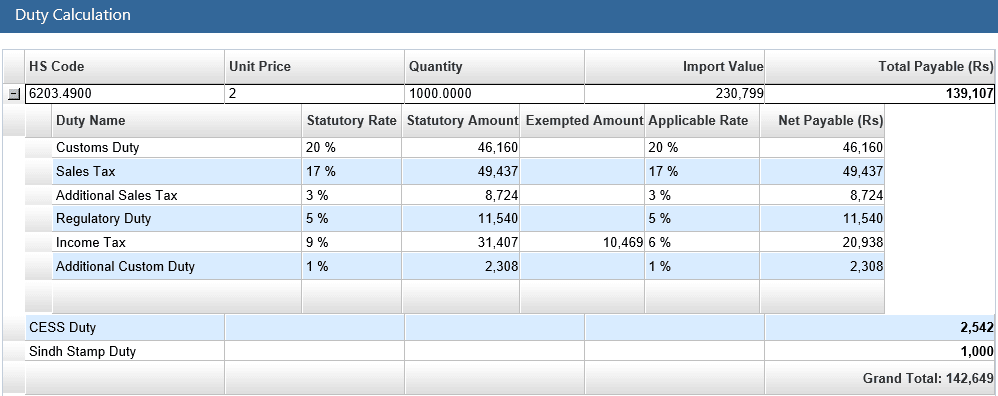# How to Calculate Customs Duties and Taxes with Simple Calculator – Live Import Fees/Charges Calculation in Pakistan

Last Updated: February 1, 2020
Category: Uncategorized

Hello friends, Here we will explain How to Calculate Customs Duties and Taxes with Simple Calculator, We learn here Import Fees/Charges Calculation and also give examples of customs duty calculation along with other taxes i.e. sales tax, income tax, regulatory duty etc.

## How to Calculate Customs Duties and Taxes with Simple Calculator – Live Import Fees/Charges Calculation in Pakistan

Calculating Import Customs Charges manually is the quite complicated process for the new trader, but here we tried to make this calculation process simple & easy.### How to Calculate Import Customs Charges (Duties & Taxes) in Pakistan

Let suppose we are the commercial importer and we want to import 1000 PCs of Denim Jeans (HS Code 6203.4900) and unit price is 2 USD Per Pc so the total value of this consignment will be 2000 US\$, here we see how can we calculate customs duties and taxes against this shipment.

### How to Determine Customs Value of your Shipment

We have to calculate customs value first because we will need this value to find out import customs duty, sales tax, and income tax etc.
 CNF Value of Cargo = 2000 US\$ Plus: Landing Charges = 20 US\$ (1% of CNF Value) Plus: Insurance = 68 US\$ Customs Value (US\$) = 2088 US\$ Exchange Rate (Rs.) = 110.5359 (Let Suppose) Customs Value (Rs.) = 230799 (2088 X 110.5359)

### 1) How to Calculate Regulatory Duty (5%)

We will add customs value, customs duty, and additional customs duty, after adding all these values simply take 17% of this calculated value.
 Customs Value (Rs.) = 230799 Regulatory Duty (Rs.) = 11540 (230799 X 5%)

### 2) Calculate Import Customs Charges Manually (21%)

Once we calculated the customs value of goods in Pak Rupees, so simply take customs value with the duty percentage of your products, In our example product is JENIM JEANS and its customs duty is 21%.

Customs Duty (Rs.) = 46160 (230799 X 20%)

As a commercial importer, we pay extra 1% Addition Customs Duty.
Additional Customs Duty = 2308 (230799 X 1%)

### 3) How to Calculate Sales Tax on Imported Goods. (20%)

We will add customs value, customs duty, and additional customs duty, and regulatory duty after adding all these values simply take 17% of this calculated value.
 Customs Value (Rs.) = 230799 Plus: Customs Duty (Rs.) = 46160 Plus: Additional Customs Duty (Rs.) = 2308 Plus: Regulatory Duty (Rs.) = 11540 Total = 290807 Sales Tax (Rs.) = 49437 (290807 X 17%)

We also pay extra 3% Addition Sales Tax, due to being commercial importer

Additional Sales Tax (Rs.) =  8724 (290807 X 3%)

### 4) Procedure to Calculate Income Tax on Imported Products (6%)

To calculate the income tax amount, simply add customs value, customs duty, and additional customs duty, sales tax, and additional sales tax, and regulatory once added all value take 6%.
 Customs Value (Rs.) = 230799 Plus: Customs Duty (Rs.) = 46160 Plus: Additional Customs Duty (Rs.) = 2308 Plus: Sales Tax (Rs.) = 49437 Plus: Additional Sales Tax (Rs.) = 8724 Plus: Regulatory Duty (Rs.) = 11540 Total = 348968 Income Tax (Rs.) = 20938 (348968 X 9%)

### Total Customs Duty & Taxes of Our Consignment Calculated

Finally! we have calculated the customs duty and other taxes such as sales tax and income tax, regulatory duty, of our consignment
 Customs Duty (Rs.) = 46160 Sales Tax (Rs.) = 49437 Additional Sales Tax (Rs.) = 8724 Regulatory Duty (Rs.) = 11540 Income Tax (Rs.) = 20938 Additional Customs Duty = 2308 Total Duty & Taxes = 139020